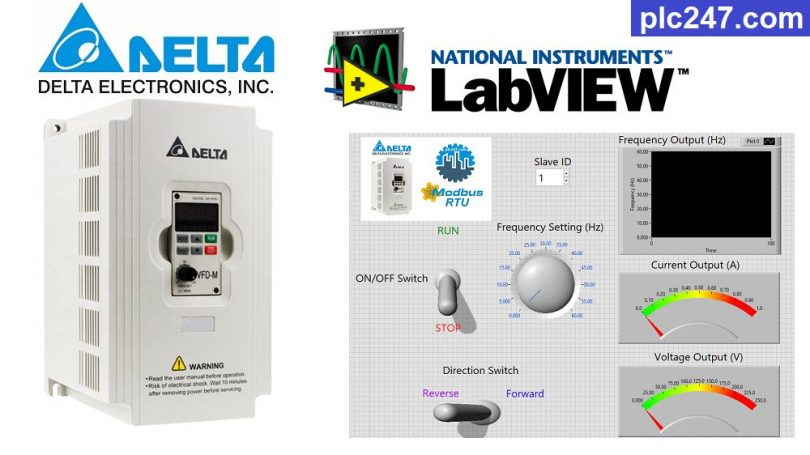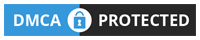# LabView “Modbus RTU” Delta VFD M TutorialWritten by

Hello all of you. Today I would like to continue to guide you to use Labview to control Delta VFD-M inverters via Modbus RTU RS485 communication.

We need to prepare a USB to RS485 converter cable. Connection diagram from PC to inverter as shown below

#### DELTA VFD-M Comunication Configuration

In addition to the basic parameters set by the motor (Frequency, Current, Voltage). We set the communication parameters as follows

+ P00 = 03 (Frequency Set by RS485)

+ P01 = 04 ( Control by RS485)

+ P88 = 01 (Slave Address)

+ P89 = 01 (Baudrate 9600bps)

+ P92 = 04 ( Mode 8/1/Even)

+ P157 = 01 (Modbus-RTU Selection)

>>> Motor Control Address = 2000 (hex) = 8192 (dec)

• Set Word 8192 = 18 >>> Motor Forward
= 34 >>> Motor Reverse
= 1 >>> Stop

>>> Frequency Set Address = 2001 (hex) = 8193 (dec)

>>> Output Frequency Address = 2103 (hex) = 8451 (dec)

+ Control Panel

+ Program

### Project Video Tutorial

======